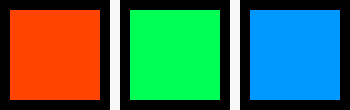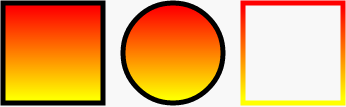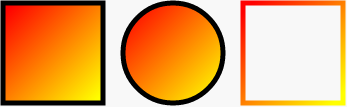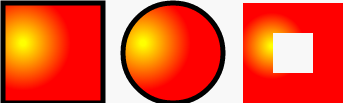DAY 10
1

Day10 - SVG fill 填色1.基本純色<rect x="50" y="50" width="100" height="100" stroke="#000" stroke-width="10" fill="#f40"></rect>
<rect x="170" y="50" width="100" height="100" stroke="#000" stroke-width="10" fill="#0f5"></rect>
<rect x="290" y="50" width="100" height="100" stroke="#000" stroke-width="10" fill="#09f"></rect>

2.漸層(之後會有篇幅詳細介紹)

<defs>
<stop offset="5%" stop-color="#F00" />
<stop offset="95%" stop-color="#ff0" />
</defs><rect x="50" y="250" width="100" height="100" stroke="#000" stroke-width="5" fill="url(#a1)"></rect>
<circle cx="220" cy="300" r="50" stroke="#000" stroke-width="5" fill="url(#a1)"></circle>
<rect x="290" y="250" width="100" height="100" stroke="url(#a1)" stroke-width="5" fill="none"></rect><defs>
<stop offset="5%" stop-color="#ff0" />
<stop offset="95%" stop-color="#f00" />
</defs>

2.1 換成垂直的漸層：<defs>
<linearGradient id="a1" x1="0%" y1="0%" x2="0%" y2="100%">
<stop offset="5%" stop-color="#F00" />
<stop offset="95%" stop-color="#ff0" />
</defs>

2.2 換成傾斜的漸層：<defs>
<linearGradient id="a1" x1="0%" y1="0%" x2="100%" y2="100%">
<stop offset="5%" stop-color="#F00" />
<stop offset="95%" stop-color="#ff0" />
</defs>

2.3 改變中心點的漸層：<defs>
<stop offset="5%" stop-color="#ff0" />
<stop offset="95%" stop-color="#f00" />
</defs>

3.圖案 ( 之後會有篇幅專門介紹 )：

<defs>
<pattern id="a2" patternUnits="userSpaceOnUse" x="0" y="0" width="30" height="30">
<rect x="0" y="0" width="25" height="25" stroke="#none" fill="#09f"></rect>
</pattern>
</defs><circle cx="220" cy="300" r="50" stroke="#000" stroke-width="5" fill="url(#a2)"></circle>

SVG 30 天 ~ 就要醬玩31